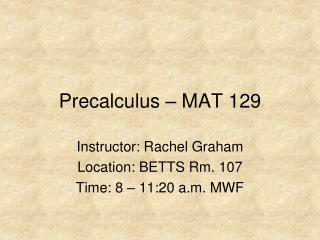DownloadDownload PresentationPrecalculus – MAT 129

# Precalculus – MAT 129

Download Presentation## Precalculus – MAT 129

- - - - - - - - - - - - - - - - - - - - - - - - - - - E N D - - - - - - - - - - - - - - - - - - - - - - - - - - -
##### Presentation Transcript

1. Precalculus – MAT 129 Instructor: Rachel Graham Location: BETTS Rm. 107 Time: 8 – 11:20 a.m. MWF

2. Chapter Ten Analytic Geometry in Three Dimensions

3. Ch. 10 Overview • The Three-Dimensional Coordinate System • Vectors in Space • The Cross Product of Two Vectors • Lines and Planes in Space

4. 10.1 – The 3-D Coordinate System • The 3-D Coordinate System • The Distance and Midpoint Formulas • The Equation of a Sphere

5. 10.1 – The 3-D Coordinate System This text book uses a right-handed system approach. Figure 10.1 on pg. 742 shows a diagram of this orientation. Note the three planes: xy, xz, and zy

6. 10.1 – Distance and Midpoint The distance between the points (x1, y1, z1) and (x2, y2, z2) is given by the formula: d = The Midpoint formula is given by:

7. 10.1 – The Equation of a Sphere The standard equation of a sphere with center (h,k,j) and radius = r is given by: (x – h)2 + (y – k)2 + (z – j)2 = r2

8. Example 1.10.1 Pg. 745 Examples 4 & 5 These are the two ways I want you to know how to do these.

9. Activities (746) 1. Find the standard equation of a sphere with center (-6, -4, 7) and intersecting the y-axis at (0, 3, 0). 2. Find the center and radius of the sphere given by: . x2 + y2 + z2 - 6x + 12y + 10z + 52 = 0

10. 10.2 – Vectors in Space • Vectors in Space • Parallel Vectors

11. 10.2 – Vectors in Space Standard form: v = v1i + v2j + v3k Component form: v = <v1,v2,v3> See all of the properties in the blue box on page 750.

12. Example 1.10.2 Write the vector v = 2j – 6k in component form.

13. Solution Example 1.10.2 <0, 2, -6>

14. 10.2 – Angle Between Two Vectors If Θ is the angle between two nonzero vectors u and v, then cos Θ = u ∙ v / ||u|| ||v||

15. Example 2.10.2 Pg. 752 Example 3 Simply following the formulas will be all you need to do.

16. 10.2 – Parallel Vectors Two vectors are parallel when one is just a multiple of the other.

17. Example 3.10.2 Pg. 752 Example 4

18. 10.2 – Collinear Points If two line segments are connected by a point and are parallel you can conclude that they are collinear points.

19. Example 4.10.2 Pg. 753 Example 5

20. 10.3 – The Cross Product of Two Vectors • The Cross Product • Geometric Properties of the Cross Product • The Triple Scalar Product

21. 10.3 – The Cross Product To find the cross product of two vectors you do the same steps as if you were finding the determinant of a matrix. Note the algebraic properties of cross products in the blue box on pg. 757.

22. Example 1.10.3 Pg. 758 Example 1 You want to leave it in i, j, k form.

23. 10.3 – Geom. Properties of the Cross Product See the blue box on pg 759. note: orthogonal means perpendicular.

24. Example 2.10.3 Pg. 759 Example 2 This is the kind of thing you will have to do again.

25. 10.3 – The Triple Scalar Product When we move up a dimension we get to a triple scalar product which is a combination of the stuff that we have learned so far. See the blue boxes on pg. 761.

26. Example 3.10.3 Pg. 761 Example 4 Pay close attention! As you should remember from determinants, these can be tricky.